C Program to Print Sum of Digit in given Number

In this tutorial you will learn about the C Program to Print Sum of Digit in given Number and its application with practical example.

C Program to Print Sum of Digit in given Number

In this tutorial, we will learn to create a C program that will Print Sum of digits in a given Number using C programming.

Prerequisites

Before starting with this tutorial we assume that you are best aware of the following C programming topics:

• Operators in C Programming.
• Basic Input and Output function in C Programming.
• Basic C programming.
• While loop in C programming.
• Conditional Statements in C programming.
• Arithmetic operations in C Programming.

Program to Print Sum of digits in a given Number

In c programming, it is possible to take numerical input from the user and Print Sum of digits in a given number with the help of a very small amount of code. The C language has many types of header libraries which has supported function in them with the help of these files the programming is easy.

With the help of this program, we can Print Sum of digits in a given number.

Output:-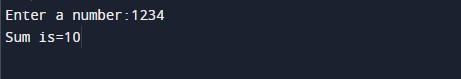In the above program, we have first initialized the required variable.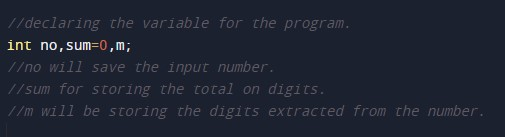• no = it will hold the integer value of the input.
• sum = it will hold the integer value for sum.
• m = it will hold the integer value.

Input number from the user.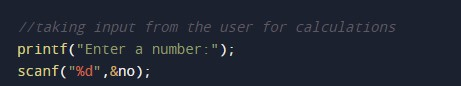Program Logic Code.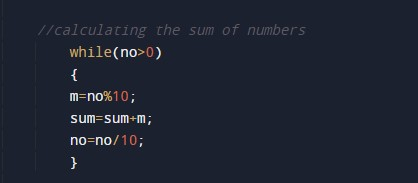Printing output for sum the digits.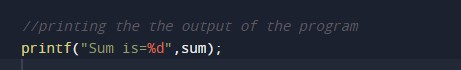In this tutorial we have learn about the C Program to Print Sum of Digit in given Number and its application with practical example. I hope you will like this tutorial.